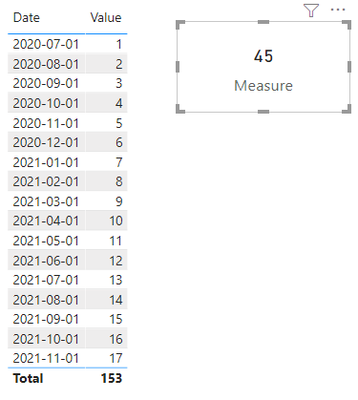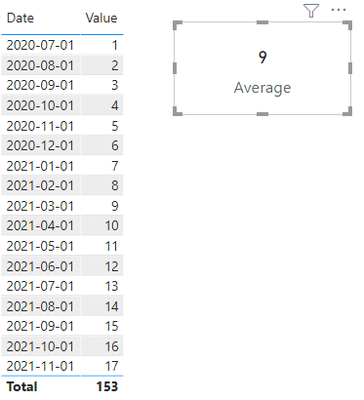cancel
Showing results for
Did you mean:

Fabric is Generally Available. Browse Fabric Presentations. Work towards your Fabric certification with the Cloud Skills Challenge.New Member

## End Of Quarter Moving Average of five previous n quarters at the closing

Hello Guys,

I am having a big issue trying to get an average of the last endofquarter of the past n quarters ( I want past 5 quarters but It could change)

I need that if the quarter doesn´t end the value should take the value only for quarters ended. I.e: If I am in November, the values should be the ones from September, June, March, December (Previous Year), September (Previous Year).

I´ve went with the pattern of moving average, mixing with the endofquarter function. But it is not working properly. Any advice on how I could workorund this issue? Or where my asumption is not right.

VAR NumOfQuarters = 5
VAR LastCurrentDate = MAX ( 'Dates'[Dates] )
VAR
Period = DATESINPERIOD ( 'Dates'[Dates], LastCurrentDate, - NumOfQuarters, QUARTER )
VAR Result =
CALCULATE (
SUMX (
VALUES ( 'Dates'[Month] ),
ENDOFQUARTER([Sales],Dates[Quarter])
),
Period
)
VAR FirstDateInPeriod = MINX ( Period, 'Dates'[Dates] )
VAR LastDateWithSales = MAX (FinalData[Date] )
RETURN
IF ( FirstDateInPeriod <= LastDateWithSales, Result)
1 ACCEPTED SOLUTIONCommunity Support

Try this,

Dax code:

``````Measure = //get total of the last endofquarter of the past 5 quarters
var _NumOfQuarters = -5
var _CurrentDate=DATE(2021,11,2)//TODAY()//If I am in 2021-11-2. you can change it later according to your needs.
var _Start= STARTOFQUARTER(DATESINPERIOD('calendar'[Date],_CurrentDate,_NumOfQuarters,QUARTER))
var _End= STARTOFQUARTER(DATESINPERIOD('calendar'[Date],_CurrentDate,1,QUARTER))
return CALCULATE(SUM(Data[Value]),FILTER(ALL(Data),Data[Date]>=_Start && Data[Date]<_End && MONTH(Data[Date]) in {3,6,9,12}))````````````Average =
var _NumOfQuarters = -5
var _CurrentDate=DATE(2021,11,2)//TODAY()//
var _Start= STARTOFQUARTER(DATESINPERIOD('calendar'[Date],_CurrentDate,_NumOfQuarters,QUARTER))
var _End= STARTOFQUARTER(DATESINPERIOD('calendar'[Date],_CurrentDate,1,QUARTER))
return Divide(CALCULATE(SUM(Data[Value]),FILTER(ALL(Data),Data[Date]>=_Start && Data[Date]<_End && MONTH(Data[Date]) in {3,6,9,12})),ABS(_NumOfQuarters))``````Best Regards,

Community Support Team _Tang

If this post helps, please consider Accept it as the solution to help the other members find it more quickly.

3 REPLIES 3Community Support

Try this,

Dax code:

``````Measure = //get total of the last endofquarter of the past 5 quarters
var _NumOfQuarters = -5
var _CurrentDate=DATE(2021,11,2)//TODAY()//If I am in 2021-11-2. you can change it later according to your needs.
var _Start= STARTOFQUARTER(DATESINPERIOD('calendar'[Date],_CurrentDate,_NumOfQuarters,QUARTER))
var _End= STARTOFQUARTER(DATESINPERIOD('calendar'[Date],_CurrentDate,1,QUARTER))
return CALCULATE(SUM(Data[Value]),FILTER(ALL(Data),Data[Date]>=_Start && Data[Date]<_End && MONTH(Data[Date]) in {3,6,9,12}))````````````Average =
var _NumOfQuarters = -5
var _CurrentDate=DATE(2021,11,2)//TODAY()//
var _Start= STARTOFQUARTER(DATESINPERIOD('calendar'[Date],_CurrentDate,_NumOfQuarters,QUARTER))
var _End= STARTOFQUARTER(DATESINPERIOD('calendar'[Date],_CurrentDate,1,QUARTER))
return Divide(CALCULATE(SUM(Data[Value]),FILTER(ALL(Data),Data[Date]>=_Start && Data[Date]<_End && MONTH(Data[Date]) in {3,6,9,12})),ABS(_NumOfQuarters))``````Best Regards,

Community Support Team _Tang

If this post helps, please consider Accept it as the solution to help the other members find it more quickly.New Member

Thank you but it isn´t working. The formula, I think, should use closingbalancequarter for using the last value of the quarter, I think I can use the moving average pattern to go back but I don´t know how to tell closingbalance quarter to only carry the closing balance I.e If it is October the last month take the previous 5 Quarterts and do the average...

Many thanks for your help... I have deadlines and I am a little bit...Super User

@pablofernandez , Try a meausre like

Rolling 5 = CALCULATE(AverageX(values(Date[Quarter Year]), calculate(lastnonblankvalue('Date'[Date]), Sum(Table[Value]))),DATESINPERIOD('Date'[Date ],MAX('Date'[Date ]),-5,Quarter))Announcements#### Power BI Monthly Update - November 2023

Check out the November 2023 Power BI update to learn about new features.#### The largest Power BI and Fabric virtual conference

130+ sessions, 130+ speakers, Product managers, MVPs, and experts. All about Power BI and Fabric. Attend online or watch the recordings.Top Solution Authors
Top Kudoed Authors
Users online (6,100)